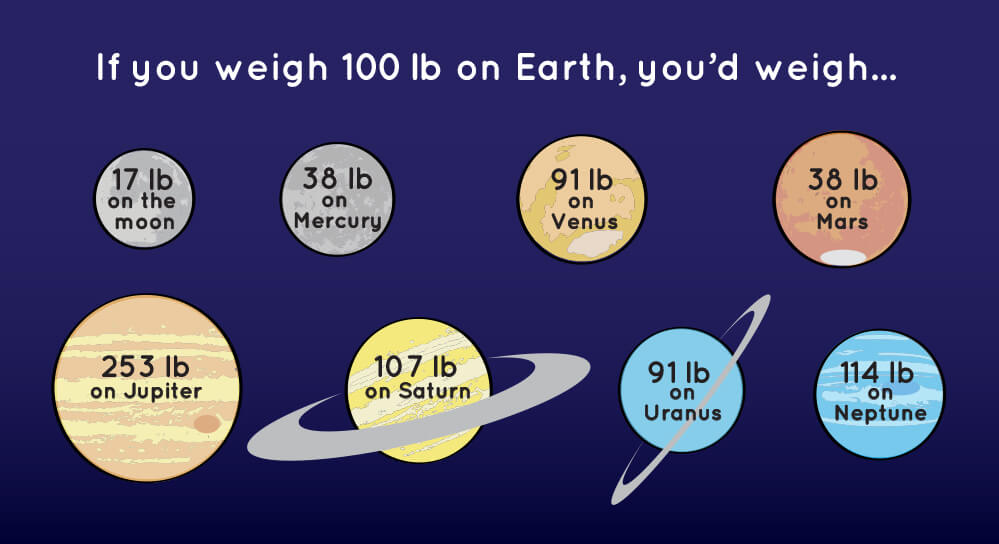# Gravity

Gravity is a fascinating phenomenon of physics that is integral to understanding the universe.  Gravity keeps Earth in orbit around the sun, and the Moon in orbit around Earth.  Any object that has mass, also has gravity. Moreover, the gravitational force between two objects is caused by two factors: mass and distance.  The gravitational force between two large objects at the same distance is greater than the gravitational force between two smaller objects.  Furthermore, the closer the distance between two objects, the greater the gravitational force.  The gravitational force between two objects can be summarized by the equation F = G * M1M2/r^2, where G is the gravitational constant, M1 is the mass of object one, M2 is the mass of object 2, and r is the distance between the two objects. As you can see, the force of gravity changes by object or planet.  Thus, an object’s weight — the force of gravity acting on an object — changes based on location, as the forces of gravity change from one planet to another (see below).  So, a person’s weight on Earth is more than their weight on Mars, but less than their weight on Jupiter.  This is because Mars is less massive than Earth, while Jupiter is more massive than Earth.  As a result, Mar’s gravitational force acting on an object on its surface is less than Earth’s, which in turn is less than Jupiter (which is more massive than Earth).  Note, a person exerts the same gravitational force on Earth (or any object they are on) as the Earth does on that person.  Yet, since the Earth is exponentially more massive than that person, your gravitational force is all but negligible on the planet, while the planet’s gravitational force keeps you from floating off into space.## 2 thoughts on “Gravity”

1.laurenegish says:

I really like the picture that you chose to include with your post. It puts the idea that “weight is the force of gravity acting upon an object” into perspective. Also I find it interesting to think Jupiter’s gravitational force is around 2.5 times that of Earth’s.

Like

2.a.brown.recluse says:

Great post content! Have you considered how the Earth’s gravity changes based on whether you are on the surface, on a mountain or deep underground in a mine?

Like